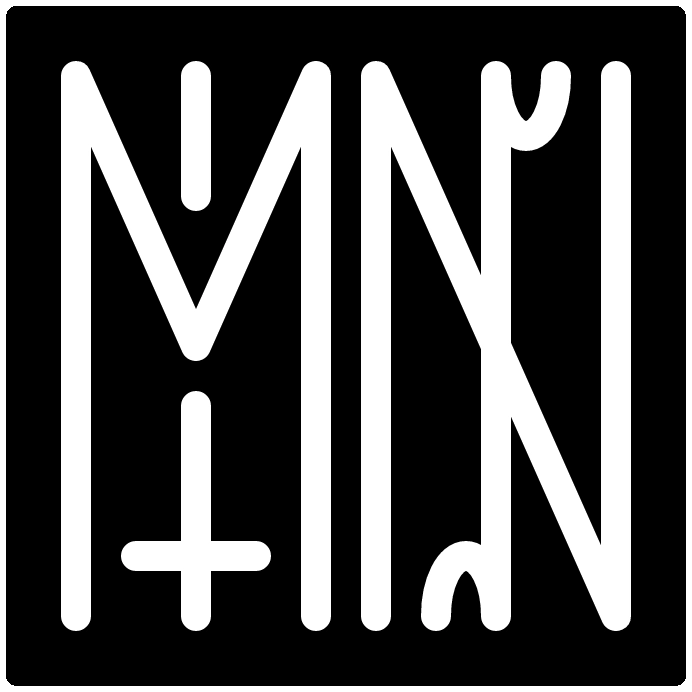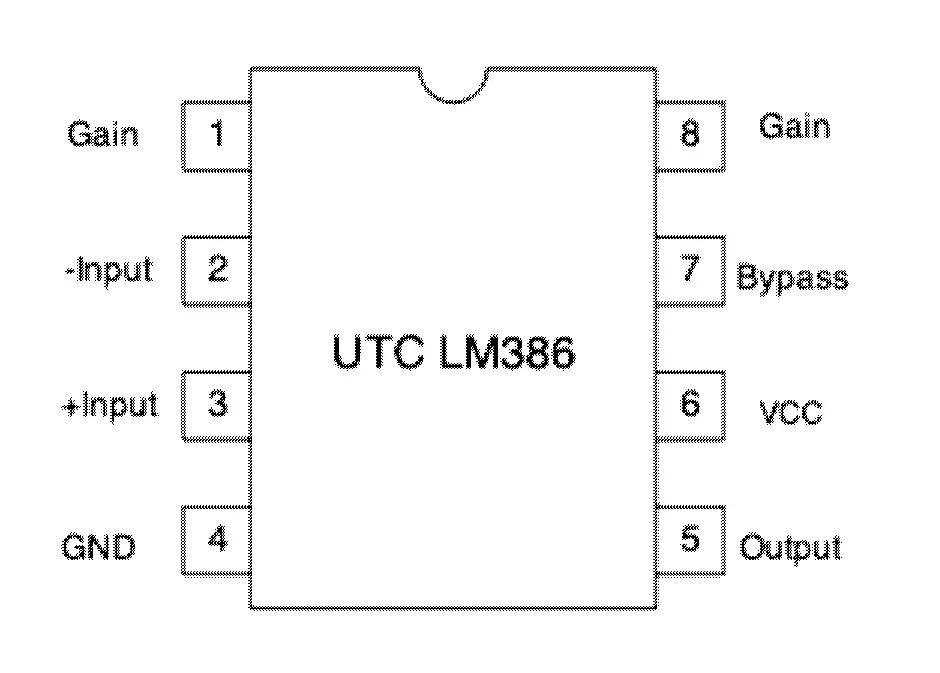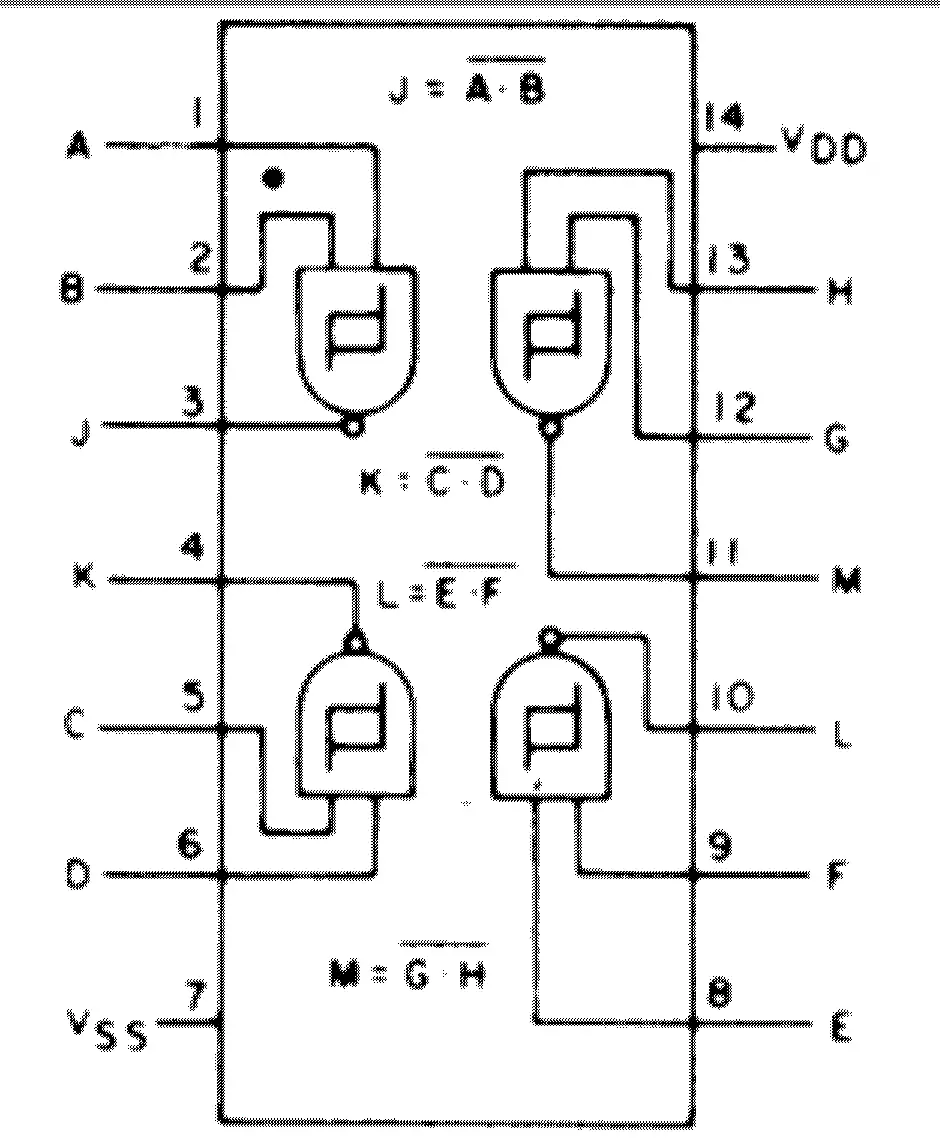directory
visual
identity
projects
lists
linguistics
misc
music# laws

## ohms law

ohm's law says that the voltage (volts; V) is equal to the current (amperes; I) divided by the resistance (ohms; R).

``````        I
V  =  _____

R
``````

all equivalent: V = I/R R = V * I I = V/R

## faraday's law

predicts how a magnetic field will interact with an electric circuit to produce a electromagnetic field, which is, electromagnetic induction

The electromotive force around a closed path is equal to the negative of the time rate of change of the magnetic flux enclosed by the path.

# units

• Voltage (volts): electric potential difference
• Ampere (amps) = electrical current
• Ohm (Ω) = electrical resistance
• Farad = capacitance of electrical potential
• K = shorthand for 1000 Ohms often

# components

## diode

one side has no resistance, other is infinite (ideally). they ensure current flows in one direction

cathode - short leg: negative end anode - long leg: positive end

light emitting diode (LED) - converts electricity into light

## resistor

resistors simply resist current! then converts current into heat.

resistors are made out of insulators and conductors. more insulators is equal to more resistance.

resistors have stripes on them. read about the electronic color code.

resistors also can serve as heating elements. if you think about a toaster, or a hair dryer, they function by means of the same mechanism as resistors.

water analogy: smaller pipe = bigger resistor

## capacitors

stores electric charge in an electric field. it is :

electrolitic capacitors are bigger - they are polarized. cermaic capacitors don't store very much charge - not polarized.

water analogy: a bucket that empties when full sorta?

## potentiometer

a three terminal resistor.

there is dial against a graphite disk the more graphite that it has to go through, the more resistance. the pivot point is connected to the middle. side pins are the opposite sides.

the terms are called:

• nose of the potentiometer (middle)
• cheek of the potentiometer (ends)

## integrated circuit

a specific set of capacitors, inductors, transistors, resistors in a circuit.

these look like small "chips". "living bug" position refers to it being with its legs down. and the flat part is at the top.

the pins on an IC have numbers like so:

``````10 9 8 7 6
| | | | |
|----------|
|\         |
| |        |
|/         |
|----------|
| | | | |
1 2 3 4 5
``````

where the left side has a marker like a half circle

# specific integrated circuits

## LM386

• low voltage audio power amplifier
• datasheet## CD4093BE

• CMOS Quad 2-Input NAND Schmitt Triggers
• datasheetlast edited: 2021.04.11